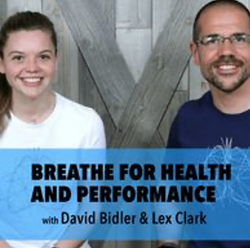Physiology First University Library: Brain Science

​The Future of Learning Begins with YouStudents: Tuition is Freecr=w_600,h_300 (2)cr=w_1360,h_740,a_cccr=w_600,h_300rs=w_1300,h_800 (5)rs=w_1300,h_800 (3)rs=w_1300,h_800 (7)rs=w_600,h_300,cg_true (3)84491012_2682664875182911_54157037931593unnamed (36)rs=w_900,h_450,cg_truePicture1Screen Shot 2021-01-20 at 10.40.17 AMrs=w_1300,h_800 (6)rs=w_1300,h_800 (2)rs=w_600,h_300,cg_true (1)unnamed (36)rs=w_1300,h_800 (1)cr=w_600,h_300 (1)Screen Shot 2021-01-20 at 10.54.33 AMcr=w_600,h_300 (2)